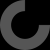Report as inappropriate

And also the rotation parameter. exRots. Appears to be the rotation angle (around Z from the original 2d object) for each point along the path.

So, the documentation, for those who are looking to know how to use this library (using path_extrude.scad), is as follows:

path_extrude(exShape=myPoints, exPath=myPath, exRots=, merge=false);`
• exShape = list of 2D points (x,y) that define the shape of the object to be extruded on a flat surface with X moving left (negative) and right (positive) and Y moving up (positive) and down (negative).
• exPath = list of 3D points (x,y,z) that define the path that the object will be extruded along.
• exRots = list of rotations around Z to apply to the original 2D object as you move along the path. Each position in this array corresponds to the same position in the exPath array. These rotations follow the 'right hand rule'.
• merge = a boolean (true, false) that tells the library to connect the start and end of the path if true.

Here's a working example that demonstrates all these parameters. Note that the shape is a pentagon with the top side elongated. The rotation list has the first element rotated 45 degrees so you can see which way positive numbers move the object.